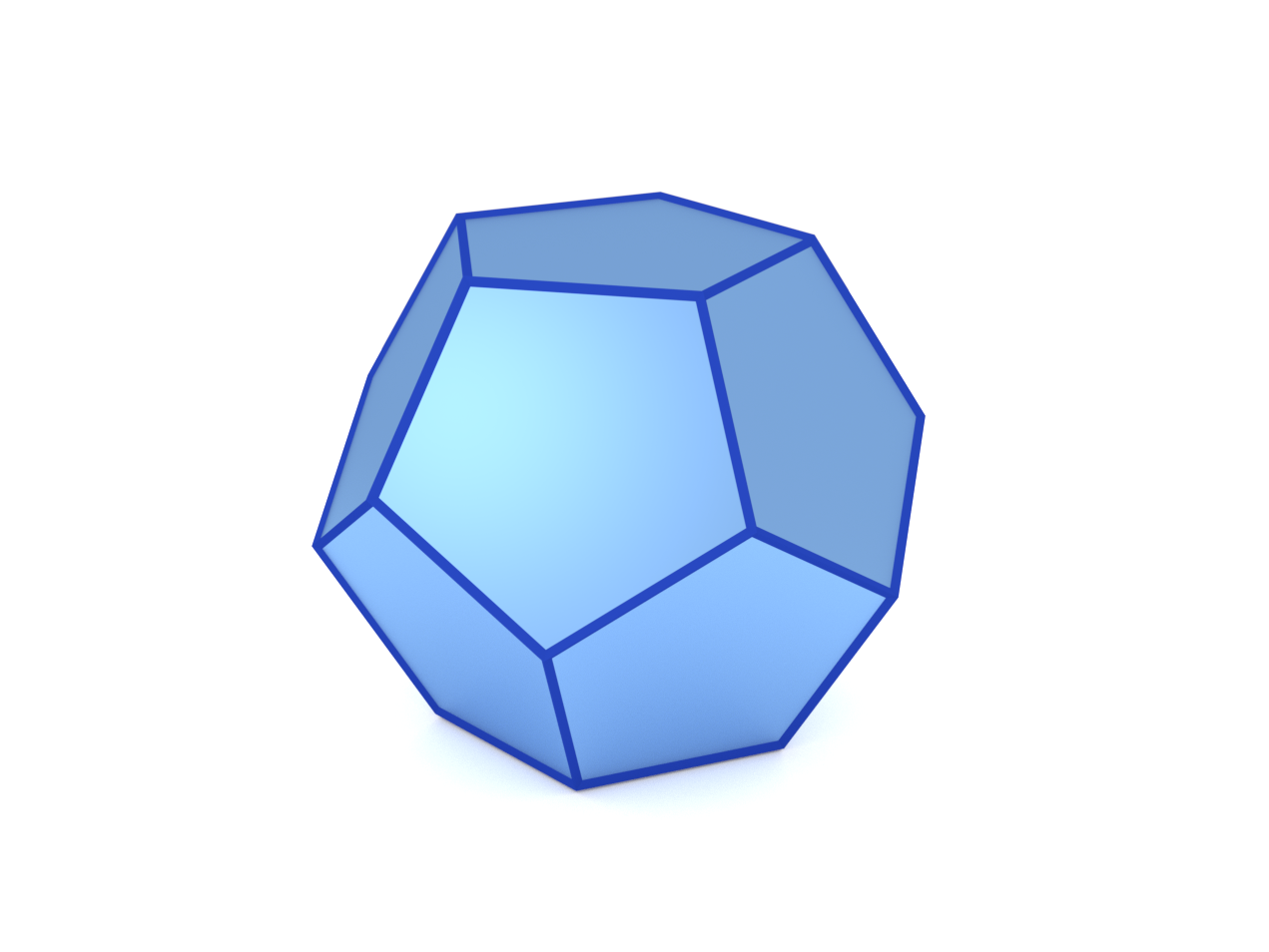### Dodecahedron

A regular dodecahedron.#### Constructor

Dodecahedron(a)


Parameters:

• a, length of the edge

#### Usage

As for any other Form factor.

#### Implementation

Class Dodecahedron inherits from the interface class IFormFactor .).

Form factor computation is based on the generic form factor of a polyhedron with inversion symmetry provided by libformfactor .

Volume has been validated against $$V= \dfrac{1}{4}(15+7\sqrt{5})a^3 \approx 7.663 \space a^3.$$

#### Example

Scattering by uncorrelated, oriented truncated dodecahedra for horizontal incidence:Generated by Examples/ff/Dodecahedron.py .

#### History

Introduced in BornAgain 1.6.